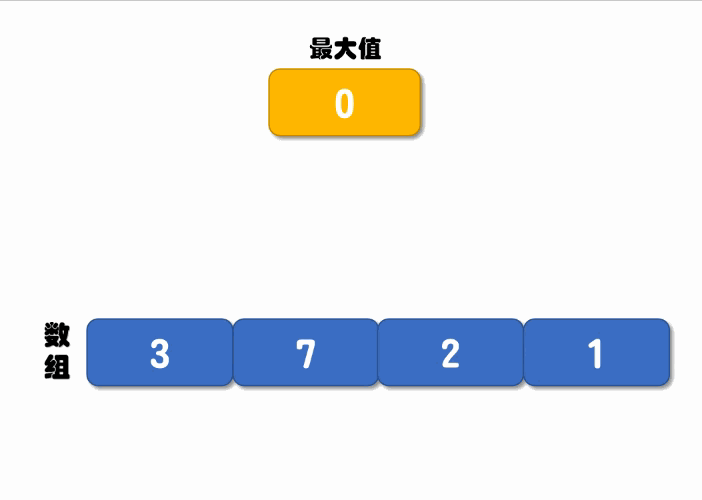# 查找数组中最大值的5种方法！(动图演示)

### 方式一：循环对比

``````public class ArrayMaxTest {
public static void main(String[] args) {
int[] arr = {3, 7, 2, 1, -4};
int max = findMaxByFor(arr); // 查找最大值
System.out.println("最大值是：" + max);
}

/**
* 通过 for 循环查找最大值
* @param arr 待查询数组
* @return 最大值
*/
private static int findMaxByFor(int[] arr) {
int max = 0; // 最大值
for (int item : arr) {
if (item > max) { // 当前值大于最大值，赋值为最大值
max = item;
}
}
return max;
}
}

### 方式二：递归对比``````public class ArrayMax {
public static void main(String[] args) {
int[] arr = {3, 7, 2, 1, -4};
int max = findMaxByRecursive(arr, 0, arr.length - 1, 0); // 根据 Collections 查找最大值
System.out.println("最大值是：" + max);
}

/**
* 根据递归查询最大的值
* @param arr  待查询数组
* @param last 最末尾的元素的下标
* @param max  （临时）最大值
* @return 最大值
*/
private static int findMaxByRecursive(int[] arr, int head, int last, int max) {
// 递归完了，返回结果
return max;
} else {
// 从后往前移动递归
return findMaxByRecursive(arr, head, last - 1, max);
} else {
max = arr[last]; // 赋最大值
// 从前往后移动递归
return findMaxByRecursive(arr, head + 1, last, max);
}
}
}
}

### 方式三：依赖 Arrays.sort() 实现

``````import java.util.Arrays;

public class ArrayMax {
public static void main(String[] args) {
int[] arr = {3, 7, 2, 1, -4};
int max = findMaxBySort(arr); // 根据 Arrays.sort 查找最大值
System.out.println("最大值是：" + max);
}

/**
* 根据 Arrays.sort 查找最大值
* @param arr 待查询数组
* @return 最大值
*/
private static int findMaxBySort(int[] arr) {
Arrays.sort(arr);
return arr[arr.length - 1];
}
}

### 方式四：根据 Arrays.stream() 实现

`stream` 是 JDK 8 新增的核心功能之一，使用它我们可以很方便的实现很多功能，比如查找最大值、最小值等，实现代码如下：

``````import java.util.Arrays;

public class ArrayMax {
public static void main(String[] args) {
int[] arr = {3, 7, 2, 1, -4};
int max = findMaxByStream(arr); // 根据 stream 查找最大值
System.out.println("最大值是：" + max);
}

/**
* 根据 stream 查找最大值
* @param arr 待查询数组
* @return 最大值
*/
private static int findMaxByStream(int[] arr) {
return Arrays.stream(arr).max().getAsInt();
}
}

### 方式五：依赖 Collections.max() 实现

``````import org.apache.commons.lang3.ArrayUtils;
import java.util.Arrays;
import java.util.Collections;

public class ArrayMax {
public static void main(String[] args) {
int[] arr = {3, 7, 2, 1, -4};
int max = findMaxByCollections(arr); // 根据 Collections 查找最大值
System.out.println("最大值是：" + max);
}

/**
* 根据 Collections 查找最大值
* @param arr 待查询数组
* @return 最大值
*/
private static int findMaxByCollections(int[] arr) {
List<Integer> list = Arrays.asList(
org.apache.commons.lang3.ArrayUtils.toObject(arr));
return Collections.max(list);
}
}

### 扩展知识：Arrays.sort 方法执行原理

``````for (int i = left, j = i; i < right; j = ++i) {
int ai = a[i + 1];
while (ai < a[j]) {
a[j + 1] = a[j];
if (j-- == left) {
break;
}
}
a[j + 1] = ai;
}

### 总结